# ICSE Class 10 Mathematics 2018 Question Paper

You have to attempt all questions from Section A and any four questions from Section B.

### Section A (40 Marks)

Question 1

(a) Find the value of ‘x’ and ‘y’ if: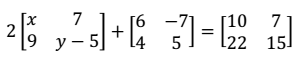(b) Sonia had a recurring deposit account in a bank and deposited Rs.600 per month for 2½ years. If the rate of interest was 10% p.a., find the maturity value of this account. 

(c) Cards bearing numbers 2, 4, 6, 8, 10, 12, 14, 16, 18 and 20 are kept in a bag. A card is drawn at random from the bag. Find the probability of getting a card which is:
(i) a prime number.
(ii) a number divisible by 4.
(iii) a number that is a multiple of 6.
(iv) an odd number. 

Question 2

(a) The circumference of the base of a cylindrical vessel is 132 cm and its height is 25 cm. Find the
(ii) volume of cylinder. (use π = 22/7) 

(b) If (k –3), (2k + 1) and (4k + 3) are three consecutive terms of an A.P., find the value of k. 

(c) PQRS is a cyclic quadrilateral. Given ∠QPS = 73°, ∠PQS = 55° and ∠PSR = 82°, calculate:
(i) ∠QRS
(ii) ∠RQS
(iii) ∠PRQ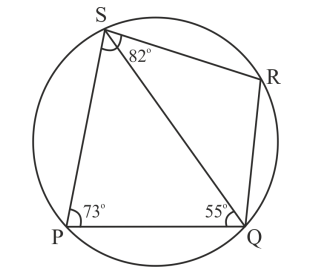Question 3

(a) If (x + 2) and (x + 3) are factors of x3 + ax + b, find the values of ‘a’ and ‘b’. 

(b) Prove that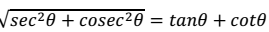(c) Using a graph paper draw a histogram for the given distribution showing the number of runs scored by 50 batsmen. Estimate the mode of the data: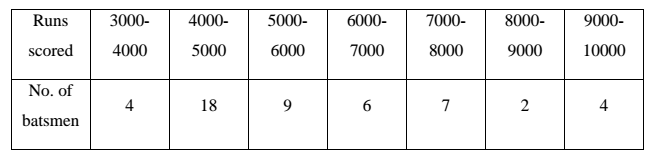Question 4

(a) Solve the following inequation, write down the solution set and represent it on the real number line: 
−2 + 10x ≤ 13x + 10 < 24 + 10x, x ∈ 𝑍

(b) If the straight lines 3x − 5y = 7 and 4x + ay + 9 = 0 are perpendicular to one another, find the value of a. 

(c) Solve x2 + 7x = 7 and give your answer correct to two decimal places. 

### Section B (40 Marks)

Question 5

(a) The 4th term of a G.P. is 16 and the 7th term is 128. Find the first term and common ratio of the series. 

(b) A man invests Rs.22,500 in Rs.50 shares available at 10% discount. If the dividend paid by the company is 12%, calculate:
(i) The number of shares purchased
(iii) The rate of return he gets on his investment. Give your answer correct to the nearest whole number. 

(c) Use graph paper for this question (Take 2 cm = 1 unit along both x and y axis).
ABCD is a quadrilateral whose vertices are A(2,2), B(2,–2), C(0,–1) and D(0,1).
(i) Reflect quadrilateral ABCD on the y-axis and name it as A'B'CD.
(ii) Write down the coordinates of A' and B'.
(iii) Name two points which are invariant under the above reflection.
(iv) Name the polygon A'B'CD. 

Question 6

(a) Using properties of proportion, solve for x. Given that x is positive: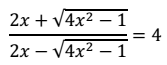(b) If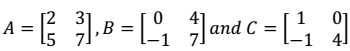, find AC + B2 – 10C. 

(c) Prove that (1 + cot θ - cosec θ)(1 + tan θ + sec θ) = 2 

Question 7

(a) Find the value of k for which the following equation has equal roots. 
x2 + 4kx + (k2 − k + 2) = 0

(b) On a map drawn to a scale of 1 : 50,000, a rectangular plot of land ABCD has the following dimensions. AB = 6 cm; BC = 8 cm and all angles are right angles. Find:
(i) the actual length of the diagonal distance AC of the plot in km.
(ii) the actual area of the plot in sq km. 

(c) A(2, 5), B(–1, 2) and C(5, 8) are the vertices of a triangle ABC, ‘M’ is a point on AB such that AM : MB = 1 : 2. Find the co-ordinates of ‘M’. Hence find the equation of the line passing through the points C and M. 

Question 8

(a) Rs.7500 were divided equally among a certain number of children. Had there been 20 less children, each would have received Rs.100 more. Find the original number of children. 

(b) If the mean of the following distribution is 24, find the value of ‘a’.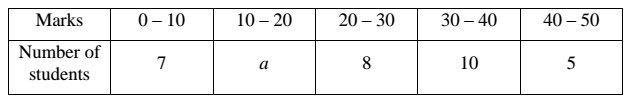(c) Using ruler and compass only, construct a ∆ABC such that BC = 5 cm and AB = 6.5 cm and ∠ABC = 120°
(i) Construct a circum-circle of ∆ABC
(ii) Construct a cyclic quadrilateral ABCD, such that D is equidistant from AB and BC. 

Question 9

(a) Priyanka has a recurring deposit account of Rs.1000 per month at 10% per annum. If she gets Rs.5550 as interest at the time of maturity, find the total time for which the account was held. 

(b) In ∆PQR, MN is parallel to QR and PM/MQ = 2/3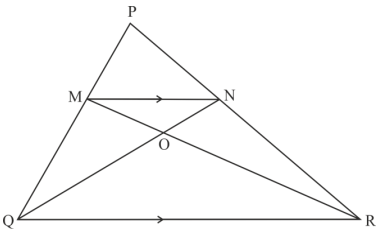(i) Find MN/QR
(ii) Prove that ∆OMN and ∆ORQ are similar.
(iii) Find, Area of ∆OMN : Area of ∆ORQ 

(c) The following figure represents a solid consisting of a right circular cylinder with a hemisphere at one end and a cone at the other. Their common radius is 7 cm. The height of the cylinder and cone are each of 4 cm. Find the volume of the solid.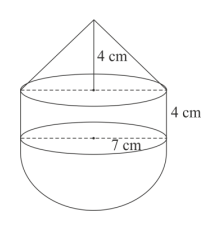Question 10

(a) Use Remainder theorem to factorize the following polynomial: 
2x3 + 3x2 − 9x − 10.

(b) In the figure given below ‘O’ is the centre of the circle. If QR = OP and ∠ORP = 20°. Find the value of ‘x’ giving reasons.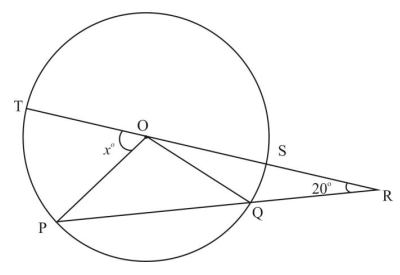(c) The angle of elevation from a point P of the top of a tower QR, 50 m high is 60° and that of the tower PT from a point Q is 30°. Find the height of the tower PT, correct to the nearest metre.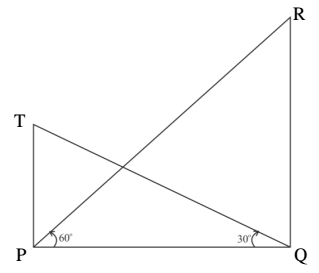Question 11

(a) The 4th term of an A.P. is 22 and 15th term is 66. Find the first term and the common difference. Hence find the sum of the series to 8 terms. 

(b) Use Graph paper for this question. 
A survey regarding height (in cm) of 60 boys belonging to Class 10 of a school was conducted. The following data was recorded: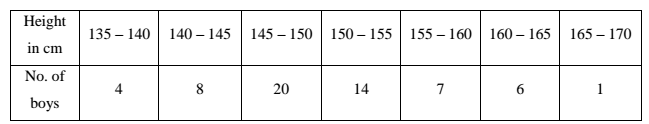Taking 2cm = height of 10 cm along one axis and 2 cm = 10 boys along the other axis draw an ogive of the above distribution. Use the graph to estimate the following:
(i) the median
(ii) lower Quartile
(iii) if above 158 cm is considered as the tall boys of the class. Find the number of boys in the class who are tall.